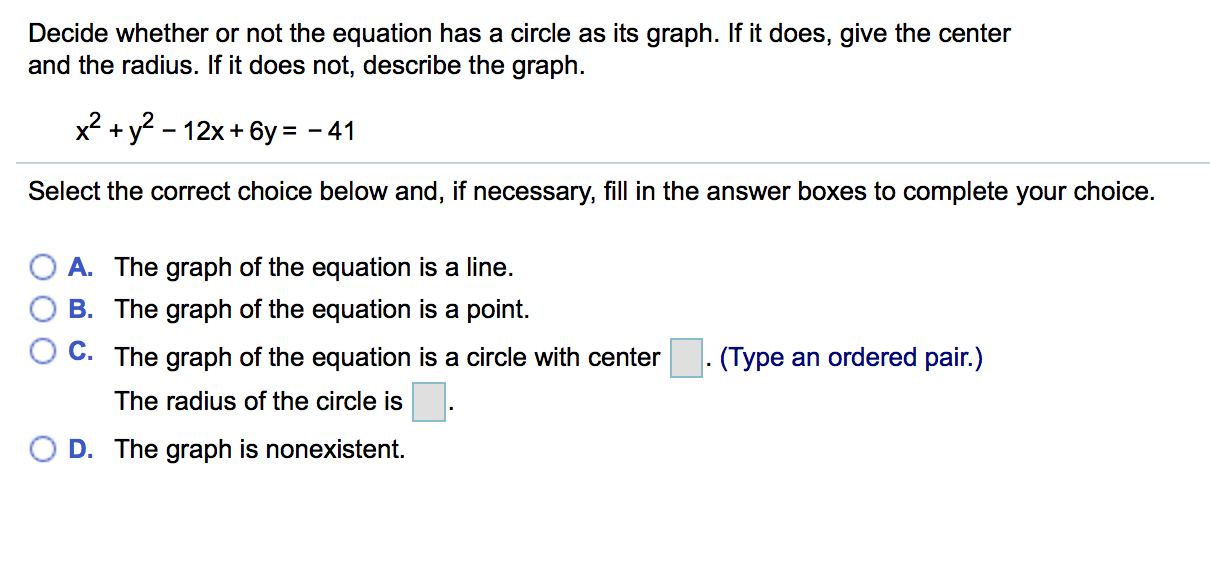# Decide whether or not the equation has a circle as its graph. If it does, give the center and the radius. If it does not, describe the graph. 2+y^-12x+6y -41 Select the correct choice below and, if necessary, fill in the answer boxes to complete your choice. OA. The graph of the equation is a line. O B. The graph of the equation is a point. C. The graph of the equation is a circle with center . (Type an ordered pair.) The radius of the circle is ( D. The graph is nonexistent.

Questionhelp_outlineImage TranscriptioncloseDecide whether or not the equation has a circle as its graph. If it does, give the center and the radius. If it does not, describe the graph. 2+y^-12x+6y -41 Select the correct choice below and, if necessary, fill in the answer boxes to complete your choice. OA. The graph of the equation is a line. O B. The graph of the equation is a point. C. The graph of the equation is a circle with center . (Type an ordered pair.) The radius of the circle is ( D. The graph is nonexistent. fullscreen

### Want to see the step-by-step answer?